# 手把手 | 教你用几行Python和消费数据做客户细分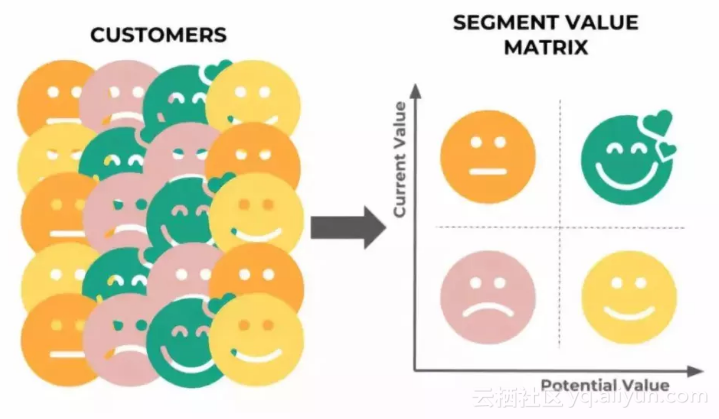●  最近消费（Recency）：他们最近一次消费是什么时候？
●  消费频率（Frequency）：他们多久消费一次、一次消费多久？
●  消费金额（Monetary）：他们消费了多少？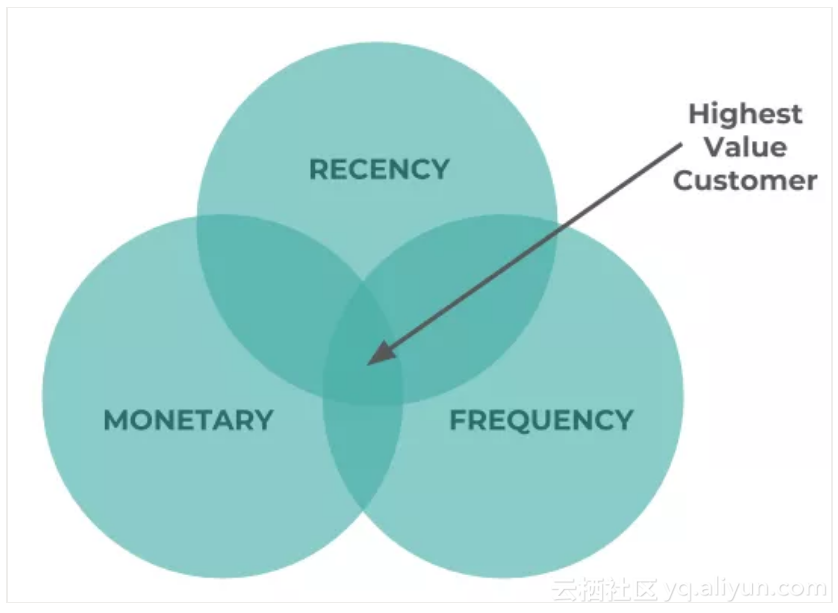RFM模型

Python实现：

import matplotlib as plt
import numpy as np
%matplotlib inline
import warnings
warnings.filterwarnings('ignore')
import pandas as pd
url = 'https://github.com/tristanga/Data-Analysis/raw/master/Global%20Superstore.xls'
df = df[(df.Segment == 'Consumer') & (df.Country == 'United States')]

df_RFM = df.groupby('Customer ID').agg({'Order Date': lambda y: (df['Order Date'].max().date() - y.max().date()).days,
'Order ID': lambda y: len(y.unique()),
'Sales': lambda y: round(y.sum(),2)})
df_RFM.columns = ['Recency', 'Frequency', 'Monetary']
df_RFM = df_RFM.sort_values('Monetary', ascending=False)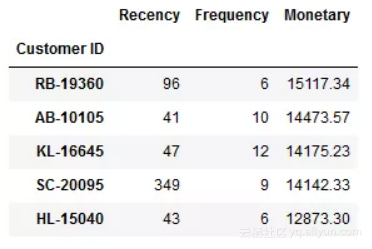# We will use the 80% quantile for each feature
quantiles = df_RFM.quantile(q=[0.8])
print(quantiles)
df_RFM['R']=np.where(df_RFM['Recency']<=int(quantiles.Recency.values), 2, 1)
df_RFM['F']=np.where(df_RFM['Frequency']>=int(quantiles.Frequency.values), 2, 1)
df_RFM['M']=np.where(df_RFM['Monetary']>=int(quantiles.Monetary.values), 2, 1)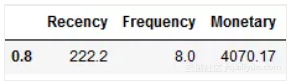# To do the 2 x 2 matrix we will only use Recency & Monetary
df_RFM['RMScore'] = df_RFM.M.map(str)+df_RFM.R.map(str)
df_RFM = df_RFM.reset_index()
df_RFM_SUM = df_RFM.groupby('RMScore').agg({'Customer ID': lambda y: len(y.unique()),
'Frequency': lambda y: round(y.mean(),0),
'Recency': lambda y: round(y.mean(),0),
'R': lambda y: round(y.mean(),0),
'M': lambda y: round(y.mean(),0),
'Monetary': lambda y: round(y.mean(),0)})
df_RFM_SUM = df_RFM_SUM.sort_values('RMScore', ascending=False)# 1) Average Monetary Matrix
 
df_RFM_M = df_RFM_SUM.pivot(index='M', columns='R', values='Monetary')
 
df_RFM_M= df_RFM_M.reset_index().sort_values(['M'], ascending = False).set_index(['M'])
 
df_RFM_M
 

 
# 2) Number of Customer Matrix
 
df_RFM_C = df_RFM_SUM.pivot(index='M', columns='R', values='Customer ID')
 
df_RFM_C= df_RFM_C.reset_index().sort_values(['M'], ascending = False).set_index(['M'])
 
df_RFM_C
 

 
# 3) Recency Matrix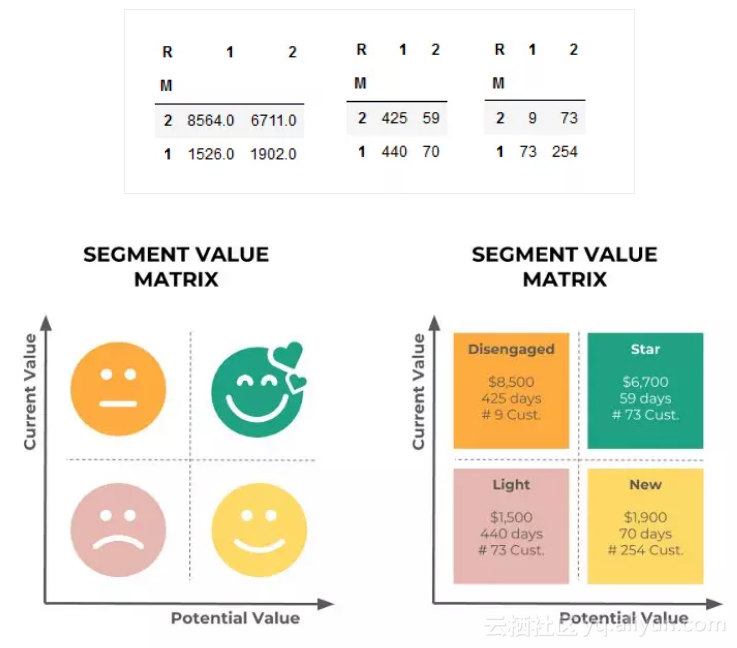“流失客户”分类中的客户人数不是很多，并且从他们身上得到的的平均收入高于“明星客户”分类。既然人数不多，从客户层面与业务部门合作对这些客户进行分析研究并制定一个留住他们的策略应该不难：给他们打电话或者直接见面，说不定就可以把他们挪到“明星客户”分类（例如，高参与度客户）。

“次要客户”分类的平均最近消费时间非常久远（超过1年，而参与度较高的客户平均来说该数据只有60至70天）。发起一些发放优惠券一类的营销活动可能能够带来新的消费，并帮助把该类客户挪至“新客户”分类（例如，高参与度客户）。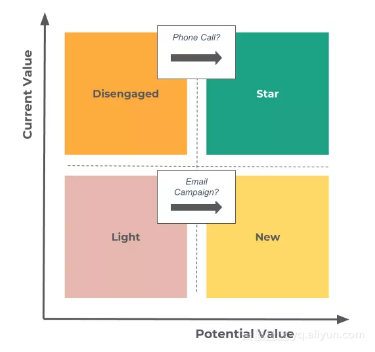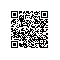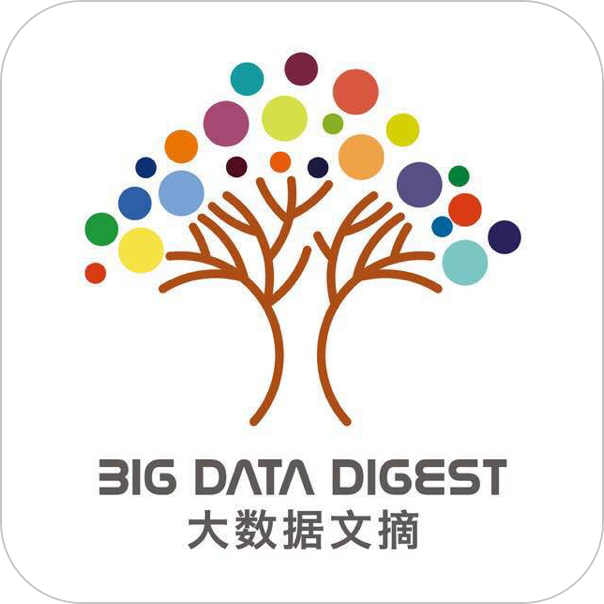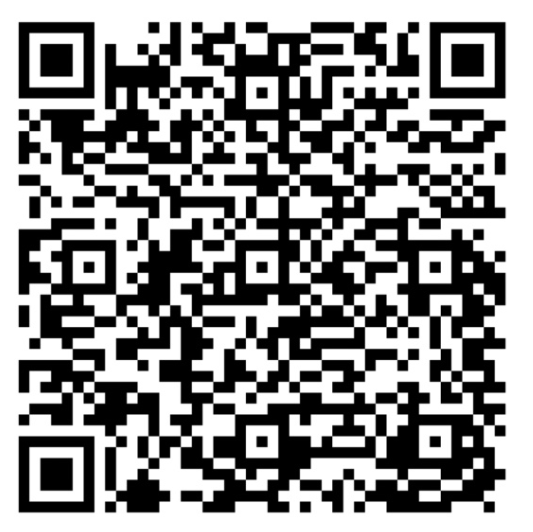使用钉钉扫一扫加入圈子
+ 订阅